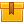# Three-Phase Circuits

## Prerequisites

The lesson requires a fundamental knowledge of electrical theory and technology.

## Description

This is the ninth lesson in the iKNOW® Electrical Theory for Troubleshooters Library. This lesson defines 3-phase AC, describes the components and operating principle of 3-phase generators, and using the formula for frequency, shows how rotor speed and the number of poles is related to frequency.

## Objectives

Define 3-phase AC. Differentiate between 3-phase and 1-phase AC. Describe the components and operating principle of a 3-phase generator. Use a sine wave to show how 3-phase voltage changes over time. Explain the relationship between frequency and rotor speed. State and apply the formula for frequency. Describe how rotor speed and the number of poles related to frequency.

## ContentThis is the ninth lesson in the iKNOW™ Electrical Theory for
Troubleshooters Library. This lesson defines 3-phase AC, describes the
components and operating principle of 3-phase generators, and using the
formula for frequency, shows how rotor speed and the number of poles is
related to frequency.

•Three-Phase Circuits

0
0 Reviews